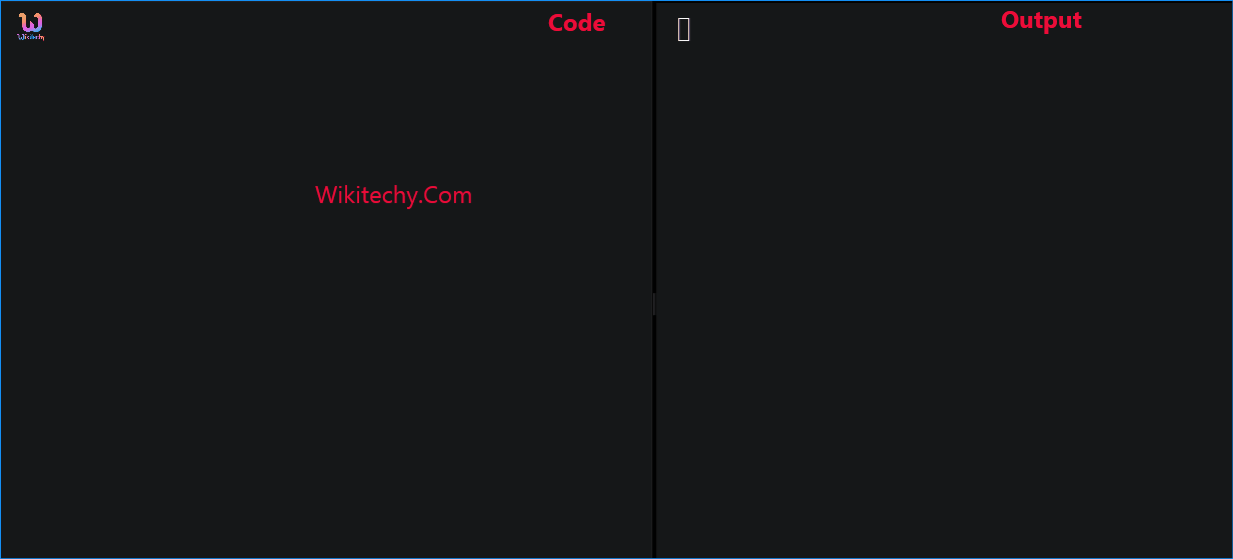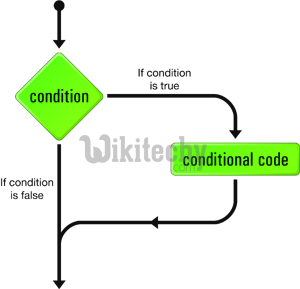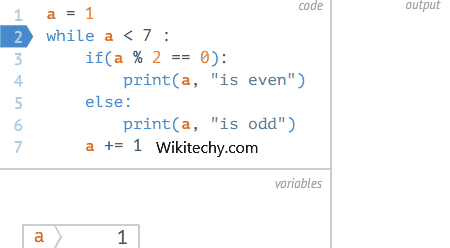# C++ If Statement | If statements - Learn C++ - C++ Tutorial - C++ programmingLearn c++ - c++ tutorial - c++ if statement - c++ examples - c++ programs

## C++ if Statement

• The if statement evaluates the test expression inside parenthesis.
• If test expression is evaluated to true, statements inside the body of if is executed.## Syntax

• The expression is called a conditional expression.
• The most basic kind of conditional branch in C++ is the if statement. An if statement takes the form:
• Here is a simple program that uses an if statement:

## Using if with multiple statements - If else statement

• Note that the if statement only executes a single statement if the expression is true, and the else only executes a single statement if the expression is false.
•learn c++ tutorials - if else loop Example

• In order to execute multiple statements, we can use a block:

## Implicit blocks

• If the programmer does not declare a block in the statement portion of an if statement or else statement, the compiler will implicitly declare one. Thus:
• Most of the time, this doesn’t matter. However, new programmers sometimes try to do something like this:
• This won’t compile, with the compiler generating an error that identifier x isn’t defined. This is because the above example is the equivalent of:
• In this context, it’s clearer that variable x has block scope and is destroyed at the end of the block.
• By the time we get to the std::cout line, x doesn’t exist

## Chaining if statements

• It is possible to chain if-else statements together:

## Nesting if statements

• It is also possible to nest if statements within other if statements :
• The above program introduces a source of potential ambiguity called a dangling else problem.
• Is the else statement in the above program matched up with the outer or inner if statement?
• The answer is that an else statement is paired up with the last unmatched if statement in the same block.
• Thus, in the program above, the else is matched up with the inner if statement.
• To avoid such ambiguities when nesting complex statements, it is generally a good idea to enclose the statement within a block.
• Here is the above program written without ambiguity:
• Now it is much clearer that the else statement belongs to the inner if statement.
• Encasing the inner if statement in a block also allows us to explicitly attach an else to the outer if statement:
• The use of a block tells the compiler that the else statement should attach to the if statement before the block.
• Without the block, the else statement would attach to the nearest unmatched if statement, which would be the inner if statement.

## Using logical operators with if statements

• You can also have if statements check multiple conditions together by using the logical operators :

## Common uses for if statements

• If statements > are commonly used to do error checking. For example, to calculate a square root.
• the value passed to the square root function should be a non-negative number:
• If statements can also be used to do early returns, where a function returns control to the caller before the end of the function.
• In the following program, if the parameter value is negative, the function returns a symbolic constant or enumerated value error code to the caller right away.
• If statements are also commonly used to do simple math functionality, such as a min() or max() function that returns the minimum or maximum of its parameters:
• Note that this last function is so simple, it can also be written using the conditional operator (?:):

## Null statements

• It is possible to omit the statement part of an if statement.
• A statement with no body is called a null statement, and it is declared by using a single semicolon in place of the statement. For readability purposes, the semicolon of a null statement is typically placed on its own line.
• This indicates that the use of a null statement was intentional, and makes it harder to overlook the use of the null statement.
• While null statements are rarely used in conjunction with if statements intentionally, they sometimes unintentionally cause problems.
• the following snippet:
• In the above snippet, the user accidentally put a semicolon on the end of the if statement.
• This unassuming error actually causes the above snippet to execute like this: